# Prekinder to Grade 2 Mathematics

Addition is a way of joining two or more numbers (or groups of things) together to make a bigger number (or group), which we call the total.

In this example we join two groups of two balls together to make a new group of four balls.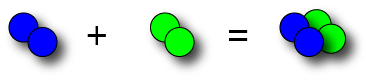We write this in numerals as:

$2 + 2 = 4$

And say it in words as:

Two plus two equals four.

Is it time for another example? This time we're going to join one group of three balls to another group of five balls: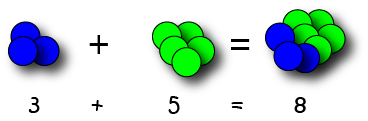In words, this says "three plus five equals eight".

## The Order Doesn't Matter

It doesn't matter which group you put down first when you're doing addition:

For example,Let's look at some other examples:

\begin{align*} 6 + 1 &= 1 + 6 = 7\\ 8 + 10 &= 10 + 8 = 18\\ 5 + 100 &= 100 + 5 = 105\\ 4 + 1000 &= 1000 + 4 = 1004 \end{align*}

To get good at addition, the best thing you can do is to get lots of practice.

Try making up some sums yourself. This is a fantastic use for brussels sprouts. Divide your brussels sprouts into groups, and add them together. Check that it doesn't matter which group you put first.

If you get in trouble for treating brussels sprouts that way, there are plenty of other things you can practise with: shells, coins, stones from the garden...just about anything you can think of.

There are lots of strategies for adding small and larger numbers in the article about tips and tricks for addition.

Let's just look at a few of them here.

#### Addition Tables for Small Numbers

Tables are really useful when you want to add smaller numbers (you have to make them too big if you want to use them to add larger numbers).

Addition tables let you look up the answers. Let's see how we'd use one to add $3$ and $4$.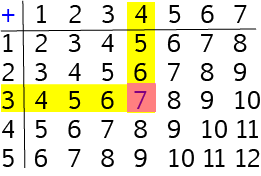• Put one finger on the row starting with $3$ and one finger on the column starting with $4$
• Run your fingers across the row and down the column until they meet.
• The place where the row starting with $3$ and the column starting with $4$ cross over is the answer. So, $3 + 4 = 7$.

There are a lot more examples of using addition tables in the article on addition tables.

#### Addition in Columns for Larger Numbers

To add larger numbers together, we write them down in columns. The hundreds, tens and ones places (and so on) of each number form the columns.

We then add each column separately. It's a good idea to add the ones column first, then the tens, then the ones, etc.

Sometimes one of the columns will spill over into the higher column, and then you'll need to adjust the total of the next column to the left.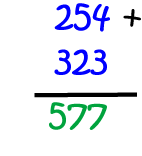### Naming the Parts of an Addition Sum

People use lots of different names for addition, or to tell you that you need to add numbers. Some words you might see are sum,plus,increase and total.

We call the numbers that we add together the addends and the result of the addition the sum or total: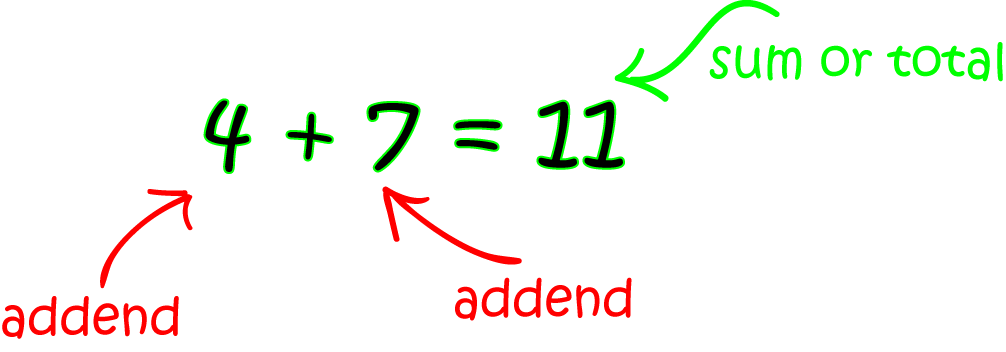### Description

This mini book covers the core of Math for Foundation, Grade 1 and Grade 2 mathematics including

1. Numbers
3. Subtraction
4. Division
5. Algebra
6. Geometry
7. Data
8. Estimation
9. Probability/Chance
10. Measurement
11. Time
12. Money
13. and much more

This material is provided free of cost for Parent looking for some tricks for their Prekinder, Kinder, Prep, Year 1 and Year 2 children

### Learning Objectives

These lessons are for kids aged 4-8 with the core objective to expose their brains to concepts of addition, subtraction, division, algebra and much more.

Author: Subject Coach
You must be logged in as Student to ask a Question.